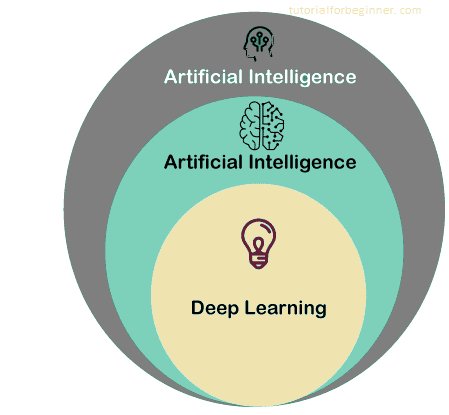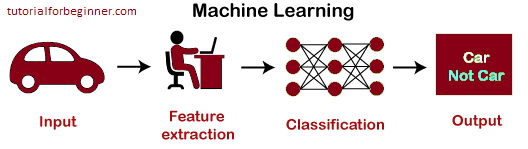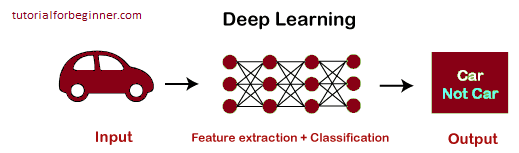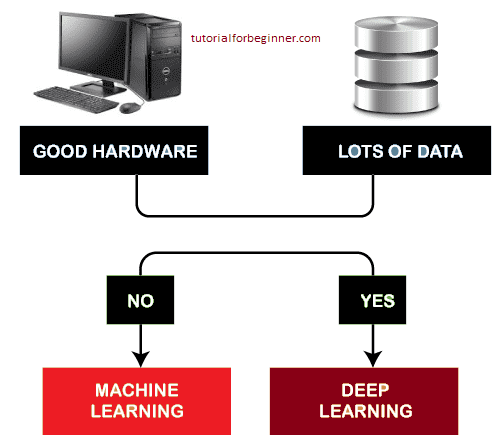# Machine Learning vs Deep Learning

In this page we will learn about Machine Learning vs Deep Learning, What is Machine Learning?, How does Machine Learning work?, What is Deep Learning?, How Deep Learning Works?, Key comparisons between Machine Learning and Deep Learning, Which one to select among ML and Deep Learning?

Data Science's two core principles and subsets of Artificial Intelligence are Machine Learning and Deep Learning. The majority of people consider machine learning, deep learning, and artificial intelligence to be interchangeable jargon. In reality, all of these words are distinct but related to one another.

We shall explore how machine learning differs from deep learning in this topic. But before we get into the distinctions, let's take a look at what machine learning and deep learning are.## What is Machine Learning?

Machine learning is a branch of artificial intelligence that allows machines to learn from previous data and complete tasks autonomously.

“Machine Leaning allows the computers to learn from the experiences by its own, use statistical methods to improve the performance and predict the output without being explicitly programmed.”

Email spam filtering, product suggestions, and online fraud detection are just a few of the common applications of machine learning.

Some useful ML algorithms are

• Decision Tree algorithm
• Naïve Bayes
• Random Forest
• K-means clustering
• KNN algorithm
• Apriori Algorithm, etc.

## How does Machine Learning work?

The workings of machine learning models can be illustrated using the example of distinguishing a cat or dog image. To do so, the machine learning model takes photographs of both cats and dogs as input, extracts various elements from the images, such as shape, height, nose, eyes, and so on, applies the classification algorithm, and predicts the outcome. Consider the following illustration:## What is Deep Learning?

Deep Learning is a subset of machine learning, or a specific type of machine learning. It works in the same manner that machine learning does in terms of technology, but with different capabilities and techniques. It is based on the functionality of human brain cells known as neurons, and it gives rise to the concept of artificial neural networks. A deep neural network, or deep neural learning, is another name for it.

Deep learning models learn and discover insights from data using multiple layers.

Self-driving cars, language translation, natural language processing, and other uses of deep learning are some of the most popular.

The following are some examples of popular deep learning models:

• Convolutional Neural Network
• Recurrent Neural Network
• Autoencoders
• Classic Neural Networks, etc.

## How Deep Learning Works?

With the same example of distinguishing cat vs. dog, we can grasp how deep learning works. The photos are fed directly to the algorithms by the deep learning model, which eliminates the need for a manual feature extraction step. The photos are passed through the artificial neural network's layers, which forecast the ultimate output.

Consider the following illustration:## Key comparisons between Machine Learning and Deep Learning

Let's look at the fundamental differences between these two words in terms of several parameters:

Parameter Machine learning Deep Learning
Data Dependency Machine learning can work with a smaller amount of data, despite the fact that it is dependent on a large number of data. Because deep learning algorithms rely heavily on a huge number of data, we must feed a significant amount of data to achieve effective results.
Execution time Machine learning algorithms take less time to train than deep learning algorithms, but they take a lengthy time to test. Deep Learning requires a long time to train a model, but only a short time to test it.
Hardware Dependencies Machine learning models can run on low-end machines since they do not require a large amount of data. To perform efficiently, the deep learning model requires a large amount of data, which necessitates the use of GPUs and hence the high-end system.
Feature Engineering Expert feature extraction is required for machine learning models before they can advance. Deep learning is an improved version of machine learning in that it does not require the development of a feature extractor for each problem; instead, it attempts to learn high-level features from data on its own.
Problem-solving approach The classic ML paradigm divides a problem into sub-parts and solves each part separately before producing the final solution. A deep learning model differs from a standard machine learning model in that it takes input for a particular problem and produces the end result. As a result, it takes an end-to-end strategy.
Interpretation of result The result for a given problem is simple to interpret. When working with machine learning, we can readily comprehend the results, which means we can understand why this outcome occurred and what the process was The interpretation of a problem's result is extremely challenging. When we use a deep learning model to solve a problem, we may get a better result than when we use a machine learning model, but we can't figure out why this particular outcome occurred and why it happened.
Type of data Data in a structured format is required by most machine learning models. Because they rely on the layers of the Artificial neural network, Deep Learning models can work with both organized and unstructured data.
Suitable for Machine learning models can be used to solve simple to somewhat complicated problems. Deep learning models are well suited to the solution of difficult issues.

## Which one to select among ML and Deep Learning?

Now that we've seen a brief overview of ML and DL, as well as some comparisons, let's look at why and which one should be used to tackle a certain problem. As a result, the flowchart below can be understood:As a result, if you have a lot of data and powerful technology, deep learning is the way to go. If none of these options are available, use the ML model to solve your problem.

b Conclusion: we may argue that deep learning is machine learning with greater capabilities and a different approach to problem solving. And deciding which one to use to tackle a particular problem is dependent on the amount of data and the problem's complexity.